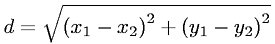Equations > Geometry > Curves and Shapes > Distance Between Two Points (2-D)

### Distance Between Two Points (2-D)Latex Code:

MathML Code:

 $d=\sqrt{{\left({x}_{1}-{x}_{2}\right)}^{2}+{\left({y}_{1}-{y}_{2}\right)}^{2}}$

MathType 5.0: